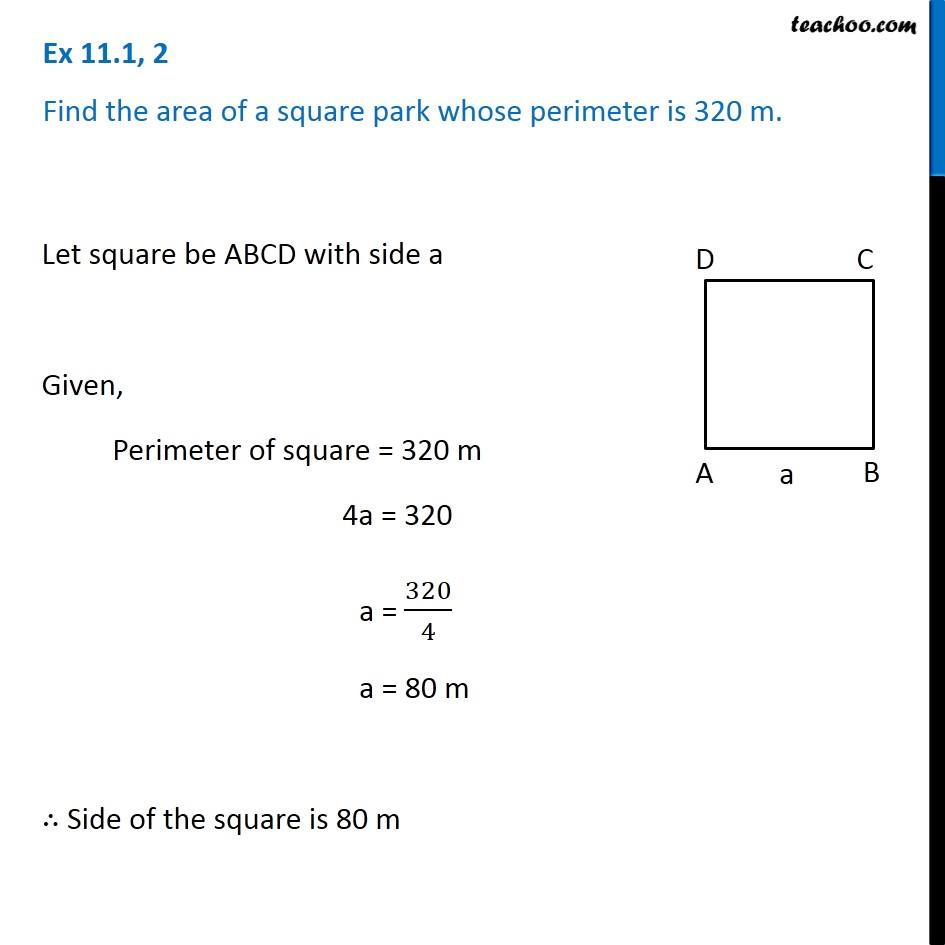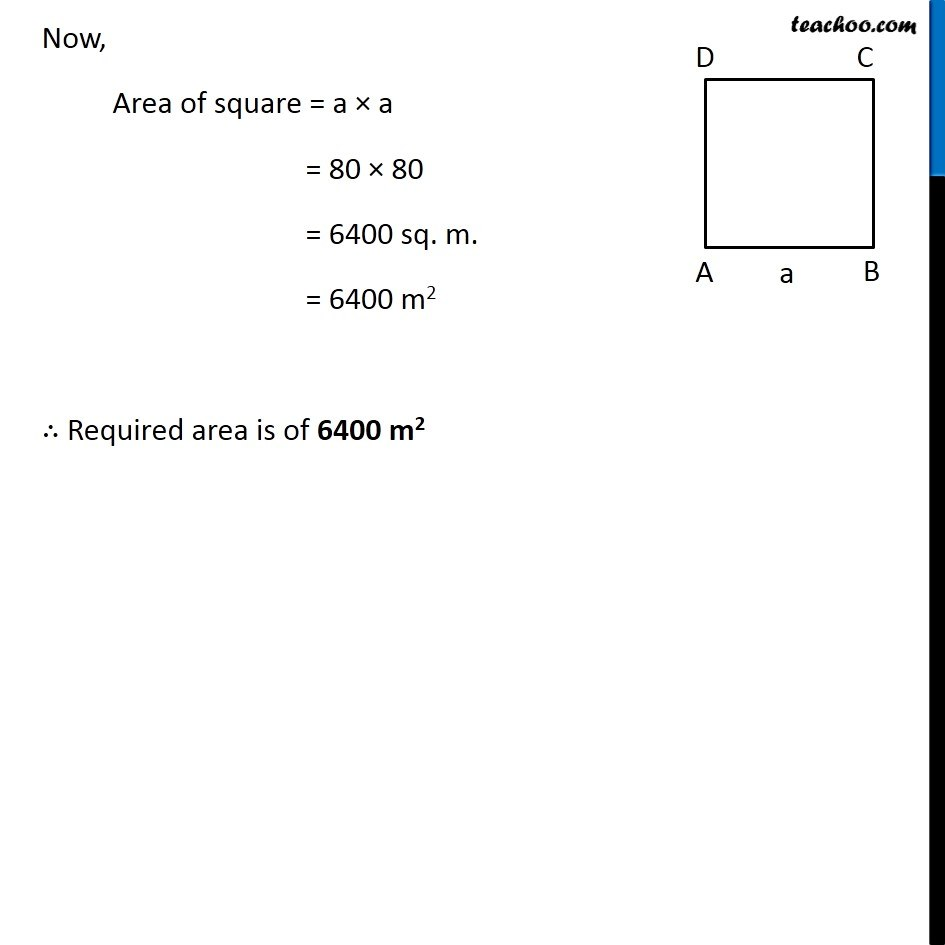Ex 11.1

Chapter 11 Class 7 Perimeter and Area
Serial order wiseGet live Maths 1-on-1 Classs - Class 6 to 12

### Transcript

Ex 11.1, 2 Find the area of a square park whose perimeter is 320 m. Let square be ABCD with side a Given, Perimeter of square = 320 m 4a = 320 a = 320/4 a = 80 m ∴ Side of the square is 80 m Now, Area of square = a × a = 80 × 80 = 6400 sq. m. = 6400 m2 ∴ Required area is of 6400 m2